Next: 5.6.2 Simulation Approach Up: 5.6 Effect of Microstructure Previous: 5.6 Effect of Microstructure

## 5.6.1 Microstructure Generation

In order to include the grain distribution into the numerical simulations, a microstructure generation tool has been developed. Given a specific interconnect structure and providing the tool with a median grain size,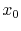, and corresponding standard deviation,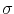, it generates a lognormal distribution of grain sizes according to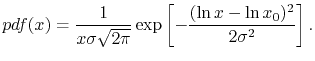(5.12)

The angles between the grain boundaries' planes and the line surface at the top follow a normal distribution,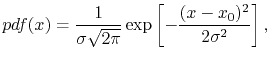(5.13)

where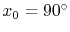is the median value of the angles. Taking a radom number,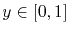, uniformly distributed, the grain sizes and grain boundary angles are determined by calculating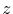, so that the inverse relation,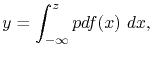(5.14)

holds. Once the grain sizes and angles are determined, the interconnect line is cut along its length by the planes which form the grain boundaries. A typical microstructure generated by such a procedure is shown in Figure 5.35. In this way, the microstructure generation tool yields a simple bamboo-like line.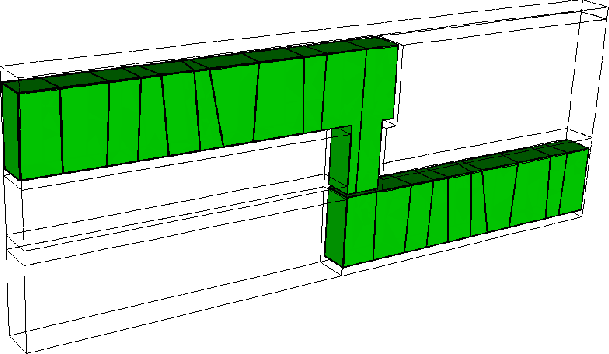Next: 5.6.2 Simulation Approach Up: 5.6 Effect of Microstructure Previous: 5.6 Effect of Microstructure

R. L. de Orio: Electromigration Modeling and Simulation